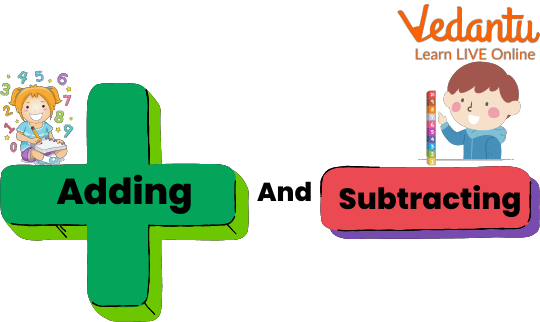Courses
Courses for Kids
Free study material
Free LIVE classes
MoreLIVE
Join Vedantu’s FREE Mastercalss

## A Brief Note on Addition and Subtraction

Can addition and subtraction be applied to a problem at the same time? Yes, addition and subtraction can be applied to the same problem at the same time. The easiest form of solving addition subtraction problems is when all the given numbers belong to the same domain. This article will help kids in learning about addition and subtraction together. The use of images and examples helps students to visualize the content and learn in a better way. Now, let us begin with our learning.

## What are Addition and Subtraction Together?

Addition and Subtraction are both arithmetic operations widely used in mathematics to solve any problem. The symbol of addition is '+' while that of subtraction is '-'. The addition is used to calculate the increase in some quantity while the subtraction is used to denote the decrease in any quantity.

## Relation Between Addition and Subtraction

The Relation between addition and subtraction is very simple and interesting. These two mathematical operations are called the inverse of each other i.e. these are opposite in nature and operation. Addition is used to add two numbers while subtraction is used to subtract two numbers. These are used to cancel each other's effects.Showing the Relation Between Addition and Subtraction

## Sum of Combinations of Addition and Subtraction

The Sum of Combinations of Addition and Subtraction is the formula which is used to solve problems involving both addition and subtraction operations at the same time. It generally prefers to solve the addition part first and then move to the subtraction part, until any bracket is not specified.

Solved examples based on addition and subtraction are given as:

Example 1: Calculate 17 + 14 - 11

Ans: Steps to calculate addition and subtraction together are given below:

• Write the given expression

• Firstly, add up the quantities i.e. 17 and 14

• Note the result i.e. 17 + 14 = 31

• Then subtract 11 from the result of the above addition i.e. 31 - 11 = 20

Thus, the result of the given addition subtraction problem is 20.

Example 2: Find the value of 18 - 11 + 1

Ans: Steps to calculate addition and subtraction together are given below:

• Write the given expression

• Firstly, add up the quantities i.e. 18 and 1

• Note the result i.e. 18 + 1 = 19

• Then subtract 11 from the result of the above addition i.e. 19 - 11 = 8

Thus, the result of the given addition subtraction problem is 8.Ans:

(a) 8

(b) 135

(c) 68

(d) 891

(e) 64

(f) 431

## Practice Problems

Some practice problems are given that need to be solved by the students on their own for clarifying the concepts deeply.

Q 1. 28 - 32 + 14

Ans: 10

Q 2. 18 + 11 - 15

Ans: 14

Q 3. 27 - 7 - 10 + 5

Ans: 15

Q 4. 12 + 38 - 14 + 10

Ans: 46

Q 5. 51 - 30 + 11 - 24

Ans: 8

Q 6. 12 - 30 + 36 - 2

Ans: 16

## Summary

Addition and Subtraction are widely used and important arithmetical operations in math. These together help in solving numerous problems easily and also makes the calculations speedy. Continuous practice of addition and subtraction sums makes the brain active and sharp by increasing memory power. This article has included all the topics related to addition subtraction, the sum of combinations formula and the relation between addition and subtraction. Feel open to putting your problems in the comments. I hope you enjoyed learning the topic.

Last updated date: 23rd Sep 2023
Total views: 92.4k
Views today: 0.92k

## FAQs on Addition and Subtraction

1. What is the additive identity of a number?

The additive identity of a number is defined as the number which when added to a number gives the number itself. Additive identity does not alter the value of a number. In general, 0 is considered the additive identity of a number i.e. 4 + 0 = 4 = 0 + 4.

2. Does subtraction follow the commutative property?

No, subtraction does not follow the commutative property because, in the case of subtraction, the order of the numbers matters i.e. difference of the numbers changes as their order changes. For example, 5 - 2 = 3 while if the order is changed i.e. 2 - 5, the result is also changed, which is equal to - 3.

3. By what term do we define the sum of subtraction of two numbers?

The term which is used to depict the sum of subtraction of two numbers is the difference. Here, the sum of subtraction denotes that one number is positive while another is negative and we take their sum which is nothing but the subtraction of these two numbers.